\$B:#>,\$N0lGU(B

\$BKhF|!":#>,\$N0lGU\$r>e\$2\$F\$\$\$^\$9!#(B
\$B:#>,0{\$`\$b\$N\$K:\$\$C\$?\$i!";29M\$K\$7\$F\$_\$F\$/\$@\$5\$\$!#(B

2022\$BG/(B6\$B7n(B

 1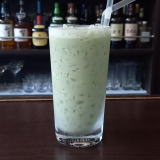88(IE2B(B 2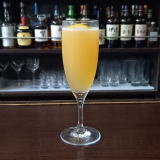(I32=70%;\0(B 3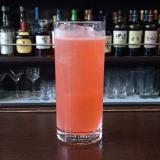(I=L_S0F(B 4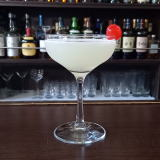(IL(0J^0(B 5 \$B5Y\$_(B 6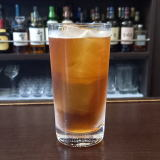(I1OZ/D%<^]<^,0(B 7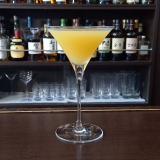(IL(/A%3*2(B 8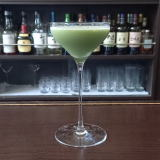(I8^X0]%L(0Y=^(B 9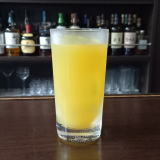(I<^,]8^Y(B 10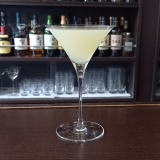(I:]A0@(B 11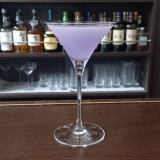(IJ_0L*8D%WL^(B 12 \$B5Y\$_(B 13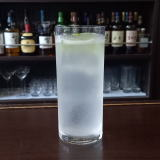(I<^]%X/70(B 14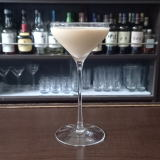(IL^W3]%M^YM^/D(B 15 \$B5Y\$_(B 16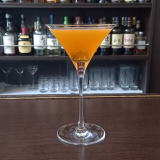(ID/J_0(B 17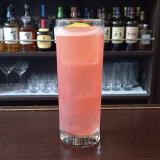(I6XL+YF1%ZSH0D^(B 18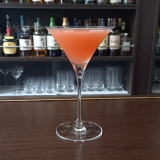(IF-0V08(B 19 \$B5Y\$_(B 20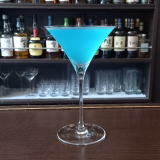(IL^Y0%O]C^0(B 21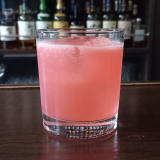(I12=%L^Z260(B 22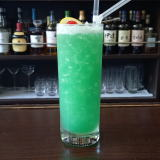(IL^Y0%J\2(B 23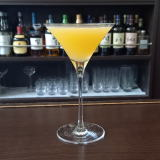(IL^[0D^3*2%;0=D(B 24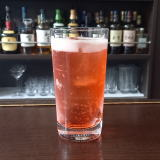(I6]J_X%?0@^(B 25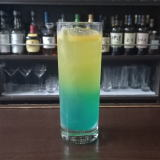(I6XL+YF1%D\2W2D(B 26 \$B5Y\$_(B 27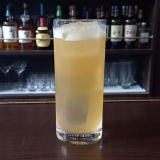(I90L^Y8^WQ(B 28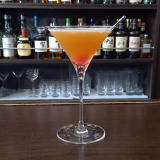(I20=D%2]C^(1(B 29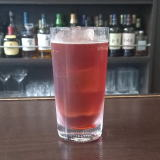(ICH<0%\YB(B 30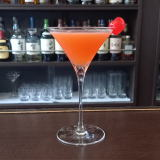(I3^(0E=(B